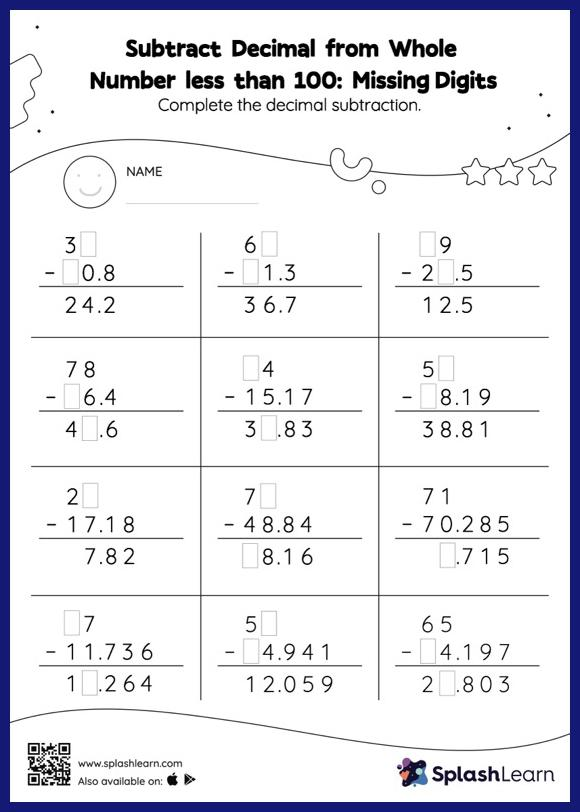# Subtract Decimal from Whole Number less than 100: Missing Digits Worksheet

Home > Subtract Decimal from Whole Number less than 100: Missing DigitsThis worksheet strengthens students' accuracy and strategic flexibility while they subtract decimal from whole number less than 100. In this worksheet, students subtract decimals by aligning the decimal points and using zero as a placeholder. They then use the relationship between addition and subtraction to find the missing number in the subtract decimal from whole number less than 100 worksheet. In this worksheet, students practice solving problems using the column method. This method is especially helpful with problems involving bigger multi-digit numbers as the format provides an easy structure to follow.